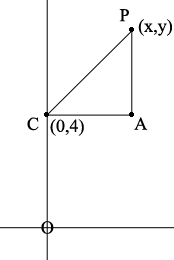SEARCH HOMEMath Central Quandaries & QueriesQuestion from mhd, a student: Complete the equation of the circle centered at(0,4) with radius 3 ---------------------------------------------- =0Hi,

I drew a diagram with $C(0,4)$ the centre of the circle and $P(x,y)$ an arbitrary point in the planeI drew a vertical line through $P$ and a horizontal line through $C,$ intersecting at $A.$

The point $P$ is on the circle with centre $C$ and radius 3 if and only if the distance from $P$ to $C$ is 3. What is the distance from $C$ to $A?$ What is the distance from $A$ to $P?$ What is the distance from $P$ to $C?$ What does Pythagoras theorem tell you about the triangle $PCA?$

PennyMath Central is supported by the University of Regina and The Pacific Institute for the Mathematical Sciences.